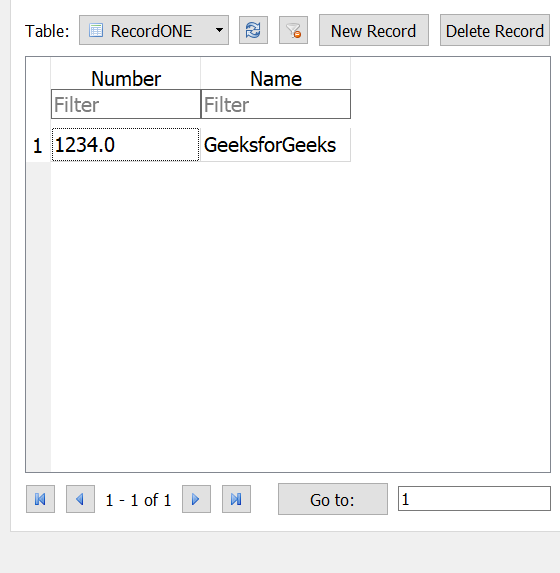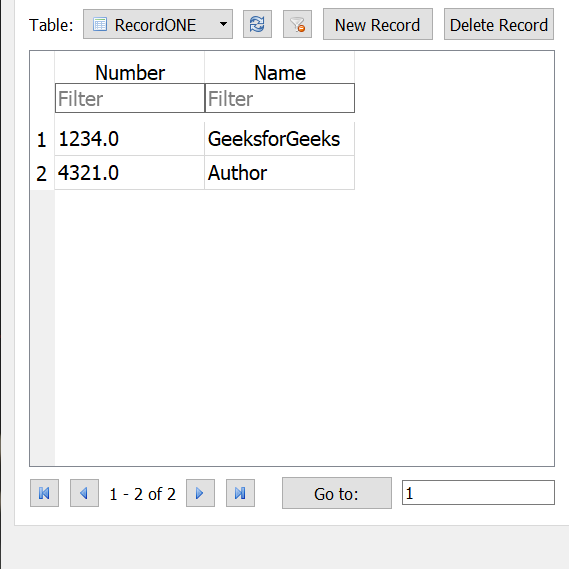Open In App

# Inserting variables to database table using Python

In this article, we will see how one can insert the user data using variables.
Here, we are using the sqlite module to work on a database but before that, we need to import that package.

`import sqlite3`

To see the operation on a database level just download the SQLite browser database.
Note: For the demonstration, we have used certain values but you can take input instead of those sample values.
Steps to create and Insert variables in database
Code #1: Create the database

## Python3

 `conn ``=` `sqlite3.connect(``'pythonDB.db'``)``c ``=` `conn.cursor()`

Explanation:
We have initialised the database pythonDB.py. This instruction will create the database if the database doesn’t exist. If the database having the same name as defined exist than it will move further. In the second statement, we use a method of sqlite3 named cursor(), this help you to initiate the database as active.
Cursors are created by the connection cursor() method, they are bound to the connection for the entire lifetime and all the commands are executed in the context of the database session wrapped by the connection.

Code #2: Create table

## Python3

 `def` `create_table():``    ``c.execute(``'CREATE TABLE IF NOT EXISTS RecordONE (Number REAL, Name TEXT)'``)`

Explanation:
We have created a function create_table. This will help you to create table if not exist, as written in the query for SQLite database. As we have initiated the table name by RecordONE. After that we pass as many parameters as we want, we just need to give an attribute name along with its type, here, we use REAL and Text.

Code #3: Inserting into table

## Python3

 `def` `data_entry():``    ``number ``=` `1234``    ``name ``=` `"GeeksforGeeks"``    ``c.execute(``"INSERT INTO RecordONE (Number, Name) VALUES(?, ?)"``,``                                                  ``(number, name))` `    ``conn.commit()`

Explanation:
Another function called data_entry. We are trying to add the values into the database with the help of user input or by variables. We use the execute() method to execute the query. Then use the commit() method to save the changes you have done above.

Code #4: Method calling and Close the connection.

## Python3

 `create_table()``data_entry()` `c.close()``conn.close()`

Explanation:
We normally use the method call, also remember to close the connection and database for the next use if we want to write error-free code because without closing we can’t open the connection again.
Let’s see the complete example now.
Example:

## Python3

 `import` `sqlite3` `conn ``=` `sqlite3.connect(``'pythonDB.db'``)``c ``=` `conn.cursor()` `def` `create_table():``    ``c.execute(``'CREATE TABLE IF NOT EXISTS RecordONE (Number REAL, Name TEXT)'``)` `def` `data_entry():``    ``number ``=` `1234``    ``name ``=` `"GeeksforGeeks"``    ``c.execute(``"INSERT INTO RecordONE (Number, Name) VALUES(?, ?)"``, (number, name))``    ``conn.commit()` `create_table()``data_entry()` `c.close()``conn.close()`

Output:Inserting one more value using data_entry() method.

## Python3

 `def` `data_entry():``    ``number ``=` `4321``    ``name ``=` `"Author"``    ``c.execute(``"INSERT INTO RecordONE (Number, Name) VALUES(?, ?)"``, (number, name))``    ``conn.commit()`

Output: